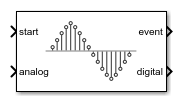Convert analog signal on ADC input pin to digital signal

• Library:
• SoC Blockset / Peripherals

•## Description

The ADC Interface block simulates the analog-to-digital conversion (ADC) of a hardware board. The input analog signal gets sampled and converted into a representative digital value. A start event message signals the block to sample the input analog voltage signal. When the conversion completes, the block emits the digital representation of the analog signal and sends an event to a Task Manager block. At this point, a connected task can execute with the new ADC sample.

## Ports

### Input

expand all

Specify an event signal to start the sampling and measurement of the analog input port signal.

Data Types: `rteEvent`

Speicy an Input analog voltage signal to convert into a digital measurement.

Data Types: `double` | `single`

### Output

expand all

This port sends the ADC Interface input signal data as a message to the msg input port of the ADC Read block.

Data Types: `SoCData`

This port sends a message at each analog to digital signal conversion event. This output connects to the input of the Task Manager block to execute the associated event-driven task after executing the ADC event.

Data Types: `rteEvent`

## Parameters

expand all

An input analog signal can be represented in digital values in the form of 12 or 16 bits. The minimum value of an analog signal that can be represented in 1 bit is called resolution. One bit represents the minimum voltage resolution measurable by the ADC. The minimum voltage resolution can be determined using the following equation:

`$\Delta {V}_{\mathrm{min}}=\frac{{V}_{ref}}{{2}^{n}}$`

where `n` is the Resolution (bits) and `Vref` is the Voltage reference (V) parameter values.

Example: `16`

The reference voltage determines the total voltage range that the ADC can convert into a digital value without saturating. Any voltage signal higher than this value produces the maximum possible value that can represented by the Resolution (bits) parameter.

Example: `3.3`

Specify the time required for the ADC to capture the input voltage during sampling.

Example: `200e-9`

Specify the required time to convert the physical voltage sample to the digital representation and output the value.

Example: `20e-9`

### External Websites

Introduced in R2020b

## SupportGet trial now